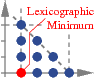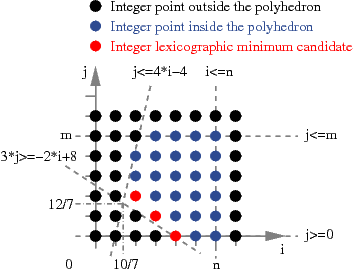What's new?

• August 2nd 2009: PIP/PipLib 1.4.0 is provided in the Download Section.
• August 2nd 2009: Page update, including new Google Groups for discussions, see the Docs Section.
• February 21th 2006: PIP/PipLib 1.3.6 is provided in the Download Section.
• November 9th 2005: PIP/PipLib 1.3.4 is provided in the Download Section.

What's PIP/PipLib?

PIP/PipLib is the well known Paul Feautrier's parametric integer linear programming solver. PIP is a software that finds the lexicographic minimum (or maximum) in the set of integer points belonging to a convex polyhedron. The very big difference with well known integer programming tools like lp_solve or CPLEX is the polyhedron may depend linearly on one or more integral parameters. If the user asks for a non integral solution, PIP can give the exact solution as an integral quotient. The heart of PIP is the parametrized Gomory's cuts algorithm followed by the parameterized dual simplex method. The PIP Library (PipLib for short) was implemented to allow the user to call PIP directly from his programs, without file accesses or system calls. The user only needs to link his programs with C libraries.

 Polyhedron to Study ContextNo parameter context. PIP will give all the solutions corresponding to all the possible cases according to the parameter values: ```if (7*n >= 10) { if (7*m >= 12) { (i = 2, j = 2) } if (2*n+3*m >= 8) { (i = -m-(m div 2)+4, j = m) } }``` With parameter context. It is possible to fully or partially define the parameter values. Then PIP will give only the solutions for the possible cases. For instance with the context: ```m >= n n >= 5``` there is only one possible solution: `(i = 2, j = 2)`

PIP stands for Parametric Integer Programming. PIP/PipLib is known to be quite stable (the project began and is active since 1988) and fast. It is used in many projects, mostly but not exclusively in automatic optimizing/parallelizing compilation (e.g. to compute data dependences).

Disclaimer